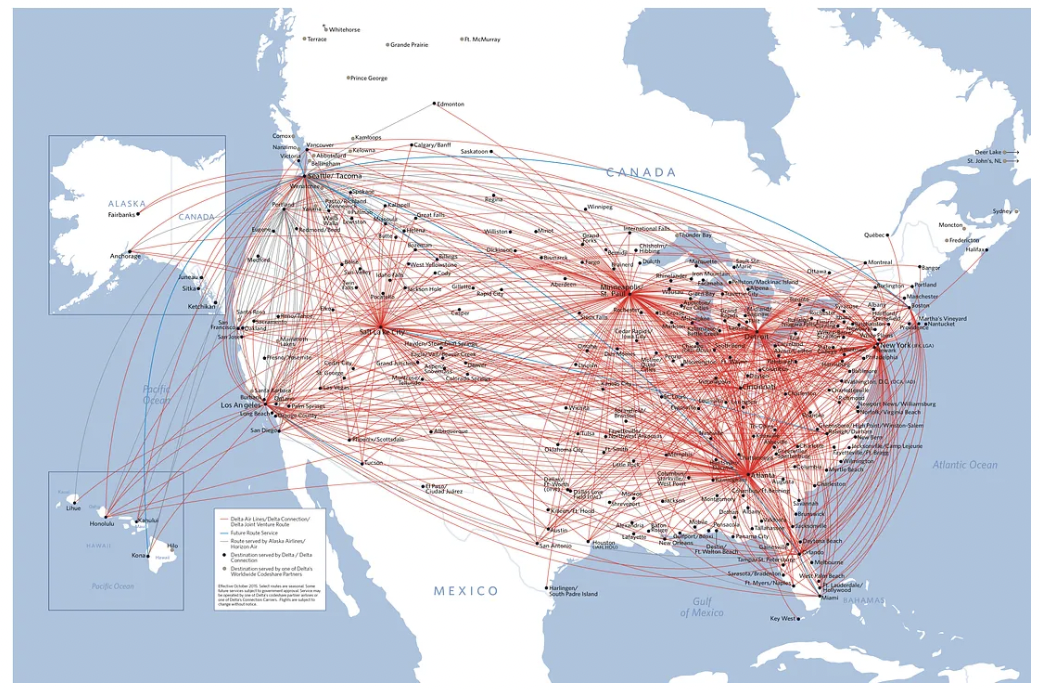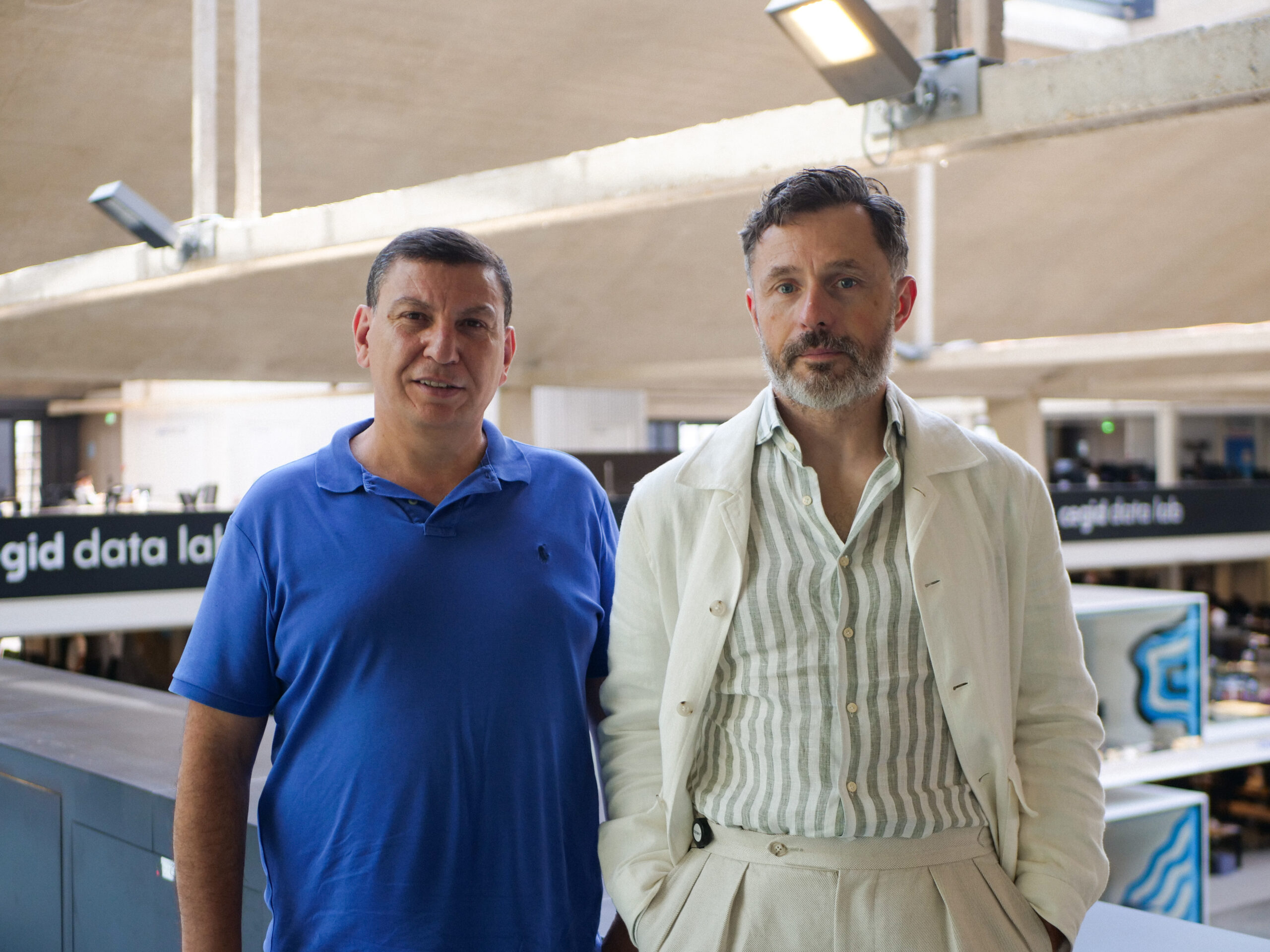# (I Can’t Get No) SATisfaction

By
Henri de Boutray
June 23, 2022
7 minutesProblem-solving is a cornerstone of our society as it allows us to create complex processes that make our lives easier. Improving those processes should probably not be an end in itself (efficiency for the beauty of efficiency), but better processes may lead to positive outcomes (efficiency for reduced energy consumption, simpler processes to understand, etc…). Amongst the problems, we are confronted with every day (conscientiously or not), discrete problems are omnipresent. The present article is on a specific class of those problems called SAT problems.

SAT or boolean SATisfiability problems are problems encoded as logic formulas. The goal is to find a value for the variables of the formula such that it evaluates to True. We say that those values satisfy the formula, and when such a set exists, the formula is satisfiable (it is not always the case!). Having the ability to solve SAT problems also enables the solving of many other discrete problems. When looking for a reason to want to solve SAT problems, we can first see that SAT is a very complex problem to solve (called an NP-complete problem) [Coo71], which makes it a great theoretical study subject to analyze fundamental notions concerning quantum advantage (in particular the likely differences between the P, NP and quantum equivalent classes of complexity). But they are much more than that.

Most discrete optimization problems can be encoded as SAT problems, which makes the SAT solvers extremely useful tools. Here is some examples of areas where SAT solvers are used:

• automated-testing (model checking), for software but not only [SBS96; BCCZ99];
• automated/semi-automated theorem proving and formal software verification [DLL62; Sta94; BFMP11];
• Electrical Design Automation (EDA): formal equivalence checking [PK00] or FPGA routing [NSR02];
• cryptoanalysis [TID20];
• various discrete optimization problems [LAK+14].

These problems are usually huge in terms of the number of variables and formula size, which implies an important need for efficient solvers.

Solving more efficiently those problems using quantum computing would then enable us to use the quantum advantage for many discrete problems.

## Good candidates for quantum computing

Many theoretical algorithms have already been exhibited (more than 400 references split into more than 60 categories of algorithms centralized in the quantum algorithm zoo [Jor21]), some of them have been run on quantum processors, but the real promise of breakthrough results thanks to quantum computing is still just that: a promise. In this context, SAT problems are especially interesting because of the huge size of the problems. Indeed, quantum computing is all about gaining a complexity advantage over classical computing: because of the nature of complexity comparison, the quantum advantage only gets bigger as the size of the systems grows. This is the reason why SAT problems are such good candidates: real-life example are typically using several thousand variables and exponentially more clauses. They are easy to check but because of the number of variables, it is extremely long to brute-force a solution.

An SAT problem is most often given as a logic formula in the Conjunctive Normal Form (CNF). A CNF is the conjunction of clauses, i.e. a set of clauses that must all be true conjointly (at the same time) for the whole formula to be true for some value of the variables (we say satisfiable). These clauses are themselves disjunction of literals, sets of literals that can be disjointly true for the clause to be true. Finally, each literal is either a variable or the negation of a variable. Concretely, a CNF would look like the formula below.

The SAT problems being in the NP-complete complexity class, are classically hard to solve. A brute-force search requires going through 2ⁿ elements if there are n variables. Since there are often around 10³ variables for this kind of problem, 2¹⁰⁰⁰ solutions need to be examined. With the current rate of computing (exascale, 10¹⁸ operations per second [Hin18]), this would take around 10²⁷⁵ years. Using a quantum search algorithm may transform these questions from unthinkable to possible.

## The known, the plan, and the promising

As they often are, this previous short presentation of a complex subject was a bit reductive. Indeed, as said previously, SAT solvers are used in day-to-day life, so naturally, SAT problems are solvable in less than 10²⁷⁵ years. Smarter than brute-force solutions exist, but they are not guaranteed to finish in a reasonable time so they are most often equipped with a timeout kill switch. These solvers are estimated to solve a problem in a time in O(1.329…ⁿ) (a short heads-up complexity theory is used a lot to evaluate quantum advantage, if you are not familiar with it, a good place to start is [this] section of the corresponding Wikipedia article).

In comparison, if we manage to create an oracle for this problem, we could use Grover’s algorithm [Gro96] to solve it with complexity in O(√N)=O(1.41…ⁿ) (the quantum circuit running the algorithm has n qubits and N=2ⁿ base states). And here is the first speed bump, as discussed in [Amb05], Grover’s algorithm alone in not sufficient to leverage a quantum advantage over the already existing algorithms that are cores to SAT solvers. This is not the end of the line though, by combining the current classical algorithms with Grover’s, we could obtain a quadratic acceleration, having complexity in O(1.153…ⁿ).

This idea of using a quantum computers to solve SAT problems was already thought of by users of Qiskit, giving us the following solution present in their documentation:

Note here that the SAT problem is encoded in the sat_cnf variable, see their GitHub for more information. But as mentioned, even if this is interesting that this solution exists, it is not faster than classical as is.

Other leads have also been explored: by nesting quantum search, Cerf et al.managed to gain an exponential speedup [CGW00], and by using a very different method altogether, Bian et al. managed really recently to use a Quantum Annealer to explore the space of possibilities by encoding the problem as an Izing model characterizing the quantum annealer [BCM+20].

Finally, de Beaudrap et al. managed to encode SAT problems into ZH-calculus — a diagrammatic quantum language taking its roots in category theory — and use its rewrite rules to give tools to simplify SAT formulas [dKM21]. This may be an interesting lead as it means that SAT formulas may be encoded as quantum circuits, but how to use those circuits to solve the SAT problems is left to be determined.

All these options are quite promising and may enable us to solve complex problems using quantum computers. And this is where our aim at ColibrITDto create a platform to solve various problems unreachable for classical computers is at!

## References

[Amb05] A. Ambainis. Quantum search algorithms. arXiv:quant-ph/0504012, 2005.
[BCCZ99] A. Biere, A. Cimatti, E. Clarke, and Y. Zhu. Symbolic Model Checking without BDDs. In W.R. Cleaveland, editor, Tools and Algorithms for the Construction and Analysis of Systems, Lecture Notes in Computer Science, pages 193–207, Berlin, Heidelberg, 1999. Springer.
[BCM+20] Z. Bian, F. Chudak, W. Macready, A. Roy, R. Sebastiani, and S. Varotti. Solving SAT (and MaxSAT) with a quantum annealer: Foundations, encodings, and preliminary results. Information and Computation, 275:104609, 2020.
[BFMP11] F. Bobot, J.-C. Filliâtre, C. Marché, and A. Paskevich. Why3: Shepherd Your Herd of Provers. In Boogie 2011: First International Workshop on Intermediate Verification Languages, page 53, 2011.
[CGW00] N. J. Cerf, Lov K. Grover, and Colin P. Williams. Nested Quantum Search and NP-Hard Problems. Applicable Algebra in Engineering, Communication and Computing, 10(4–5):311–338, 2000.
[Coo71] S. A. Cook. The complexity of theorem-proving procedures. In Proceedings of the Third Annual ACM Symposium on Theory of Computing, STOC ’71, pages 151–158, New York, NY, USA, May 1971. Association for Computing Machinery.
[dKM21] N. de Beaudrap, A. Kissinger, and K. Meichanetzidis. Tensor Network Rewriting Strategies for Satisfiability and Counting. Electronic Proceedings in Theoretical Computer Science, 340:46–59, 2021.
[DLL62] M. Davis, G. Logemann, and D. Loveland. A machine program for theorem-proving. Communica- tions of the ACM, 5(7):394–397, 1962.
[Gro96] L. K. Grover. A Fast Quantum Mechanical Algorithm for Database Search. In Proceedings of the Twenty-eighth Annual ACM Symposium on Theory of Computing, STOC ’96, pages 212–219, New York, NY, USA, 1996. ACM.
[Hin18] J. Hines. Genomics Code Exceeds Exaops on Summit Supercomputer, 2018.
[Jor21] S. Jordan. Quantum Algorithm Zoo. Technical report, 2021.
[LAK+14] Y. Li, A. Albarghouthi, Z. Kincaid, A. Gurfinkel, and M. Chechik. Symbolic optimization with SMT solvers. In Proceedings of the 41st ACM SIGPLAN-SIGACT Symposium on Principles of Programming Languages, pages 607–618, San Diego California USA, 2014. ACM.
[NSR02] G.-J. Nam, K.A. Sakallah, and R.A. Rutenbar. A new FPGA detailed routing approach via search-based Boolean satisfiability. IEEE Transactions on Computer-Aided Design of Integrated Circuits and Systems, 21(6):674–684, 2002.
[PK00] V. Paruthi and A. Kuehlmann. Equivalence checking combining a structural SAT-solver, BDDs, and simulation. In Proceedings 2000 International Conference on Computer Design, pages 459–464, 2000.
[SBS96] P. Stephan, R.K. Brayton, and A.L. Sangiovanni-Vincentelli. Combinational test generation using satisfiability. IEEE Transactions on Computer-Aided Design of Integrated Circuits and Systems, 15(9):1167–1176, 1996.
[Sta94] G. Stalmarck. System for determining propositional logic theorems by applying values and rules to triplets that are generated from boolean formula, 1994.
[TID20] M. Trimoska, S. Ionica, and G. Dequen. A SAT-Based Approach for Index Calculus on Binary Elliptic Curves. In Progress in Cryptology-AFRICACRYPT 2020, pages 214–235, Cairo, Egypt, 2020.Henri de Boutray